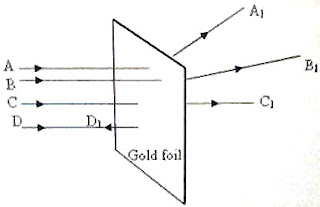## Pages

`“Life is like riding a bicycle.  To keep your balance you must keep moving.”–Albert Einstein`

## Sunday, March 14, 2010

### AP Physics B - Multiple Choice Practice Questions on Atomic Physics and Quantum Effects

Equations to be remembered in atomic physics and quantum effects were discussed on this blog in the post dated 23rd February 2008. Subsequently some typical multiple choice practice questions (with solution) were discussed in the posts dated 25th February 2008 and 1st March 2008, followed by a couple of free response practice questions (with model answers) in the post dated 8th March 2008. Some more multiple choice practice questions (with solution) were discussed in the post dated 12th March 2009. All these posts can be accessed by clicking on the label ‘atomic physics’ below this post.

Today we will discuss a few more multiple choice practice questions in this section:(1) A beam of α-particles is directed towards a gold foil. A, B, C, and D are the paths of α-particles incident on the gold foil (fig.) and A1, B1, C1, and D1 are the paths of the α-particles scattered by the gold foil. Pick out the correct statement:

(a) The number of α-particles in A1 will be minimum and those in C1 will be maximum

(b) The number of α-particles in A1 will be maximum and those in C1 will be minimum

(c) The number of α-particles in B1 will be minimum and those in C1 will be maximum

(d) The number of α-particles in C1 will be minimum and those in D1 will be maximum

(e) The number of α-particles in C1 will be maximum and those in D1 will be minimum

The volume of the nucleus in a an atom is very much insignificant compared to the volume of the atom. The space within an atom is mostly empty and the nucleus is often compared to a ‘fly in a cathedral’. So most of the α-particles incident on the gold foil proceed undeviated. Some of the α-particles are deviated by the Coulombian repulsion. The probability for a head on collision which makes the α-particle retrace its path is least. So the number of α-particles in C1 will be maximum and those in D1 will be minimum [Option (e)].

(2) An α-particle of mass m, charge 2e and speed v proceeds to make a head on collision with the nucleus of an atom of atomic number Z. What is the distance of closest approach of the α-particle?

(a) Ze2/4πε0mv2

(b) Ze2/πε0mv2

(c) Z2e2/πε0mv2

(d) Z2e4/πε0mv2

(e) Z2e2/4πε0mv2

When the α-particle approaches the nucleus its kinetic energy decreases because of the electrostatic repulsion and its electrostatic potential energy increases. At the distance of closest approach (d) the entire kinetic energy gets converted into electrostatic potential energy and so we have

(½) mv2 = (1/4πε0) (Ze×2e/d)

[Note that the nuclear charge is Ze].

This gives d = 4Ze2/4πε0mv2 = Ze2/πε0mv2

(3) Consider a system consisting of an electron and a photon. The photon collides with the electron and gets scattered. In this process the kinetic energy of the electron is found to be increased. Compared with the incident photon, the scattered photon has

(a) larger energy

(b) smaller speed

(c) smaller wave length

(d) smaller momentum

(e) larger speed

By the law of conservation of energy, the scattered photon has smaller energy E. The momentum of the photon is E/c where c is the speed of the photon. Since the speed c is constant, the momentum of the scattered photon must be smaller [Option (d)].

(4) Light of frequency 1.8 times the threshold frequency is incident on a photosensitive surface. If the frequency of the incident light is halved and the intensity of the light is doubled, the photoelectric current will be

(a) unchanged

(b) halved

(c) doubled

(e) zero

Photo electrons will not be emitted if the frequency of the incident light is less than the threshold frequency. Therefore, the photoelectric current will be zero for all incident intensities [Option (e)].

(5) A proton, a deuteron (nucleus of deuterium or heavy hydrogen) and an α-particle are accelerated by the same potential difference. Their velocities will be in the ratio

(a) 1:1:1

(b) 1:2:4

(c) √2:1:1

(d) 1:1:√2

(e) 1:2:2

The kinetic energy acquired is given by

(½)mv2 = qV where m is the mass, v is the velocity and q is the charge of the particle accelerated by a potential difference V.

Therefore, v = √(2qV/m).

The velocity is thus directly proportioal to √(q/m).

The ratio of velocities of the proton, deuteron and α-particle is:

1 : (1/√2) : √(2/4) = 1 : (1/√2) : (1/√2) = √2:1:1

[Remember that the deuteron has twice the mass of the proton and the same charge as the proton where as the α-particle has four times the mass of the proton and twice the charge of the proton].

(6) Light beams with photon energies 1.5 eV and 3 eV are allowed to fall in succession on a photosensitive surface of work function 1 eV. The ratio of the maximum speeds of the emitted electrons will be

(a) 1 : 2

(b) 1 : 1

(c) √2 : 1

(d) 1 : √2

(e) 1 : 4

The maximum kinetic energy of the emitted electron is equal to the difference between the energy of the incident photon and the work function of the photosensitive surface. Therefore we have

(½)mv2 = E Φ where m is the mass and v is the maximum velocity of the photo electron, E is the energy of the incident photon and Φ is the work function of the photosensitive surface.

Considering the emission by the two light beams, we have

(½)mv12 /(½)mv22 = (1.5 – 1)/(3 – 1) = 1/4

[We could substitute the photon energies and the work functions in electron volts since we require the ratio of velocities].

Therefore, the ratio of the maximum velocities of the emitted electrons is given by

v1/v2 = √(1/4) = 1/2 [Option (a)].